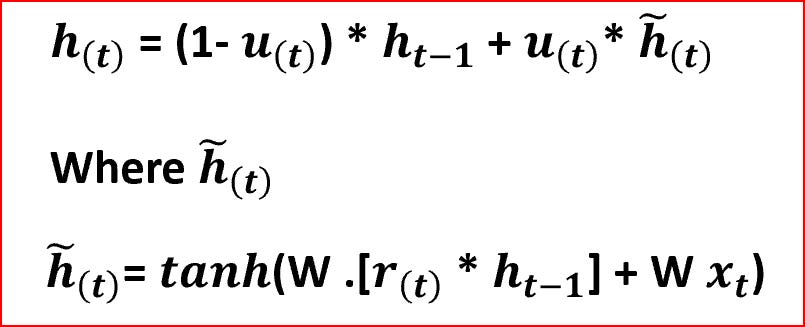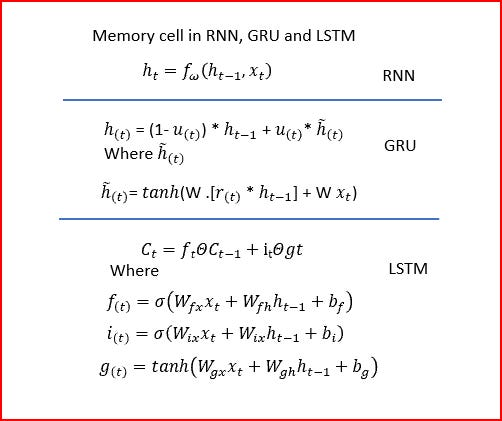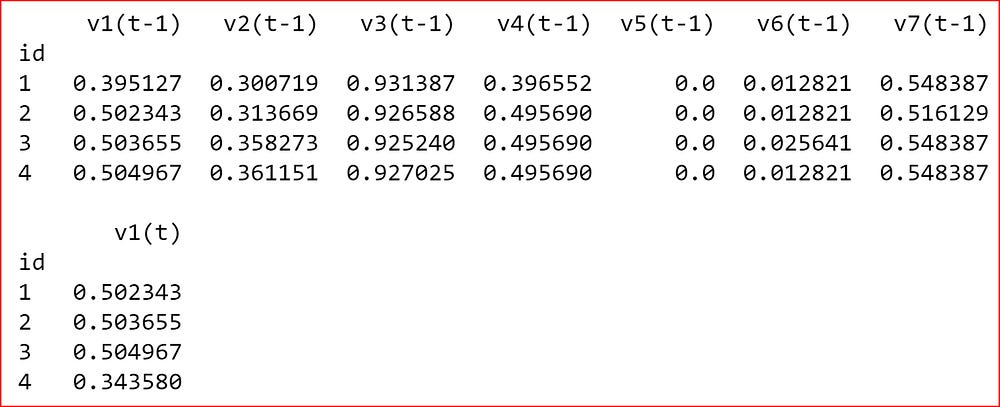Multivariate Time Series using Gated Recurrent Unit -GRU

Source: Deep Learning on Medium

In this post we will understand a variation of RNN called GRU- Gated Recurrent Unit. Why we need GRU, how does it work, differences between LSTM and GRU and finally wrap up with an example that will use LSTM as well as GRU

Prerequisites

Recurrent Neural Network RNN

Multivariate-time-series-using-RNN-with-keras

What is Gated Recurrent Unit- GRU?

• GRU is an improvised version of Recurrent Neural Network(RNN)
• Addresses the vanishing gradient problem of RNN.
• GRU is capable of learning long term dependencies

RNN are neural networks with loop to help persist information. RNN suffer from either exploding gradient or vanishing gradient issue.

What is Exploding and Vanishing gradients?

Gradients of neural network is calculated during back propagation.

With deeper neural layers in RNN and sharing weights across different RNN cell, we sum up the gradients at each time step. As gradients go through continuous matrix multiplication due to chain rule, they either shrink exponentially and have small values called Vanishing gradient or they blow up to a very large value and this is referred as Exploding gradients

How can we resolve the problem of Vanishing or Exploding gradients?

Exploding Gradient can be resolved using gradient clipping. In gradient clipping we set a pre-determined gradient threshold and when the gradients exceeds this threshold we scale the gradient to the threshold.

Vanishing Gradients is addressed either Long Short Term Memory(LSTM) or Gated Recurrent Unit(GRU).

we will discuss GRU here

How does GRU address the Vanishing Gradient problem?

For this we need to first understand how GRU works.

GRU like LSTM is capable of learning long term dependencies.

GRU and LSTM both have gating mechanism to regulate the flow of information like remembering the context over multiple time steps. Thy keep track of what information from past can be kept what can be forgotten. To achieve this GRU uses Update gate and Reset gate

What is the functionality of Update and Reset gate in remembering long term dependencies?

Update Gate

• How much of previous memory to keep around. Decide what to keep and what to throw away.
• How much of cell state you will update
• Will have a value between 0 and 1
• If the value of the update unit is close to 0 then we remember the previous state
• If value of the update unit is 1 or close to 1 then we forgot the previous value and store the new value
• Update gate acts similar to input and forget gate of LSTM

Reset Gate also known as Relevance Gate

• Reset gate decides how much of information to forget
• Allows model to drop information that is irrelevant for the future
• Determines how much of previous memory to keep around

Let’s go step by step and understand how GRU works

Step 1: Drop irrelevant information for future

Reset Gate takes the input Xt and the previous hidden state ht-1 and applies a sigmoid activation function.

Reset gate determines if the current state will have the new information or will still have the previous information

If the Reset gate has a value close to 0 then ignore previous hidden state. This means that the previous information is irrelevant and we want to drop that and store the new information.

Step 2: How much of previous memory to be stored

Update Gate takes the input Xt and the previous hidden stae ht-1 and applies a sigmoid activation function.

Update gate determines how much of previous memory to keep around, it decides what to keep and what to throw away.

If the value of the update unit is close to 0 then we remember the previous state.

Step 3: Final memory to be storedFinal hidden state or final memory

when the Reset gate rt is close to 0, previous hidden state is ignored and reset with the current input xt only.

Hidden state will drop any information that is found to be irrelevant for the future. This a compact representation.

Update gate controls how much information from the previous hidden state will carry over to the current hidden state.

If the value of the update unit is close to 0 then we remember the previous hidden state. If value of the update unit is 1 or close to 1 then we forgot the previous hidden state and store the new value

GRU has separate reset and update gates, each unit will learn to capture dependencies over different time scales. Units that learn to capture short-term dependencies will tend to have reset gates that are frequently active. Units that capture longer-term dependencies will have update gates that are mostly active

Now that we have understood the working of GRU we revisit our question of how GRU solves vanishing gradient issue.

In Vanishing gradient, the gradients become small or zero and easily vanishes.

Gating mechanism in GRU and LSTM helps resolve the vanishing gradient issue. Shutting the update gate essentially skip layers when calculating the gradient.Gates hold information in memory as long as required and update it with new information only when necessary.

Using a combination of gates either allows to pass or block, so no matter how deep our neural network or input sequence is, the network can remember the gradients .

Intuitively the error is additive instead of multiplicative and hence it is easier to keep in reasonable range. Forget gate in LSTM or Update gate in GRU help with long term dependenciesMemory cell is additive in LSTM and GRU and multiplicative in RNN

Let’s understand the commonality and difference between LSTM and GRU?

Commonality between LSTM and GRU

• LSTM and GRU both have update units with additive component from t to t + 1, which is lacking in the traditional RNN.
• LSTM unit and GRU both keep the existing content and add the new content on top of it
• Update gate in GRU and Forget gate in LSTM takes the linear sum between the existing state and the newly computed state
• LSTM and GRU addresses vanishing and exploding gradient issue present in RNN

Differences between LSTM and GRU

• GRU has two gates, reset and update gates. LSTM has three gates, input, forget and output. GRU does not have an output gate like LSTM. Update gate in GRU does the work of input and forget gate of LSTM
• GRU have fewer parameters so they are computationally more efficient and need less data to generalize than LSTM
• LSTM maintains an internal memory state c, while GRU does not have a separate memory cell
• GRU does not have any mechanism to control the degree to which its state or memory content is exposed, but exposes the whole state or memory content each time. LSTM can control how much memory content it wants to expose.

Finally we wrap up with an example that will use LSTM as well as GRU

Here I have used the Electric power consumption data set .

Importing required libraries

import pandas as pd
import numpy as np
from math import sqrt
from numpy import concatenate
from matplotlib import pyplot
from pandas import read_csv
from pandas import DataFrame
from pandas import concat
from sklearn.preprocessing import MinMaxScaler
from sklearn.preprocessing import LabelEncoder
from sklearn.metrics import mean_squared_error
from keras.models import Sequential
from keras.layers import Dense
from keras.layers import LSTM, GRU
import tensorflow as tf
from datetime import datetime

Reading the data set, parsing the dates and inferring the date format to date time. We also fill the Nan’s with 0.

parse_dates={'dt' : ['Date', 'Time']},
infer_datetime_format=True,
index_col= 0,
na_values=['nan','?'])
dataset.fillna(0, inplace=True)
values = dataset.values
# ensure all data is float
values = values.astype('float32')

Looking at the sample data from dataset

As the input features are on different scale, we need to normalize the features. We are using using Min Max scalar

# normalizing input features
scaler = MinMaxScaler(feature_range=(0, 1))
scaled = scaler.fit_transform(values)
scaled =pd.DataFrame(scaled)

Looking at the data after it is normalized

We define a function to creating the time series data set. We can specify the lookback interval and the predicted column

def create_ts_data(dataset, lookback=1, predicted_col=1):
temp=dataset.copy()
temp["id"]= range(1, len(temp)+1)
temp = temp.iloc[:-lookback, :]
temp.set_index('id', inplace =True)
predicted_value=dataset.copy()
predicted_value = predicted_value.iloc[lookback:,predicted_col]
predicted_value.columns=["Predcited"]
predicted_value= pd.DataFrame(predicted_value)

predicted_value["id"]= range(1, len(predicted_value)+1)
predicted_value.set_index('id', inplace =True)
final_df= pd.concat([temp, predicted_value], axis=1)
#final_df.columns = ['var1(t-1)', 'var2(t-1)', 'var3(t-1)', 'var4(t-1)', 'var5(t-1)', 'var6(t-1)', 'var7(t-1)', 'var8(t-1)','var1(t)']
#final_df.set_index('Date', inplace=True)
return final_df

We now create the time series dataset with looking back one time step

reframed_df= create_ts_data(scaled, 1,0)
reframed_df.fillna(0, inplace=True)

reframed_df.columns = ['var1(t-1)', 'var2(t-1)', 'var3(t-1)', 'var4(t-1)', 'var5(t-1)', 'var6(t-1)', 'var7(t-1)','var1(t)']Time series data set with one time step of look back

Splitting data set into test and train data set

# split into train and test sets
values = reframed_df.values
training_sample =int( len(dataset) *0.7)
train = values[:training_sample, :]
test = values[training_sample:, :]
# split into input and outputs
train_X, train_y = train[:, :-1], train[:, -1]
test_X, test_y = test[:, :-1], test[:, -1]

Reshaping the data set to 3D with sample size, lookback time steps and the input features.

# reshape input to be 3D [samples, time steps, features]
train_X = train_X.reshape((train_X.shape, 1, train_X.shape))
test_X = test_X.reshape((test_X.shape, 1, test_X.shape))
print(train_X.shape, train_y.shape, test_X.shape, test_y.shape)

We now create the LSTM model with 3 LSTM layers and one Dense layer. We compile the model using adam optimizer . Loss is calculated using mean absolute error(mae)

model_lstm = Sequential()

Let’s look at the LSTM model summary

model_lstm.summary()

Fitting the LSTM model

# fit network
history_lstm = model_lstm.fit(train_X, train_y, epochs=10, batch_size=64, validation_data=(test_X, test_y), shuffle=False)

We now create GRU with similar layers like LSTM

model_gru = Sequential()

Let’s look at the GRU model summary

model_gru.summary()

We can see that LSTM and GRU had the same architecture but number of parameters in LSTM is 44,971 where as GRU in GRU is 33,736. GRU is a simpler model with two gates compared to LSTM that has three gates. As GRU has less parameters it is computationally more efficient than LSTM.

Fitting the GRU model

# fit network
gru_history = model.fit(train_X, train_y, epochs=10, batch_size=64, validation_data=(test_X, test_y), shuffle=False)

For understanding how the loss varied across LSTM and GRU we plot the loss

pyplot.plot(history_lstm.history['loss'], label='LSTM train', color='red')
pyplot.plot(history_lstm.history['val_loss'], label='LSTM test', color= 'green')
pyplot.plot(gru_history.history['loss'], label='GRU train', color='brown')
pyplot.plot(gru_history.history['val_loss'], label='GRU test', color='blue')
pyplot.legend()
pyplot.show()

What did I learn while creating the model

Bad data with null values caused accuracy and loss to be NAN. To resolve that
ensure you do not have any nulls in the data

References:

Empirical Evaluation of Gated Recurrent Neural Networks on Sequence Modeling

Learning Phrase Representations using RNN Encoder–Decoder for Statistical Machine Translation

https://www.cs.toronto.edu/~guerzhoy/321/lec/W09/rnn_gated.pdf